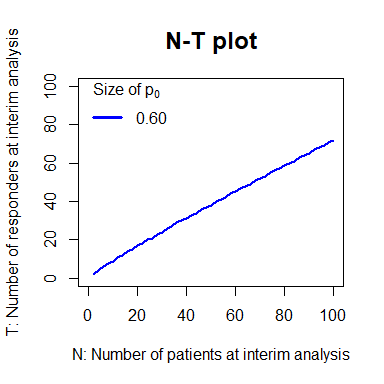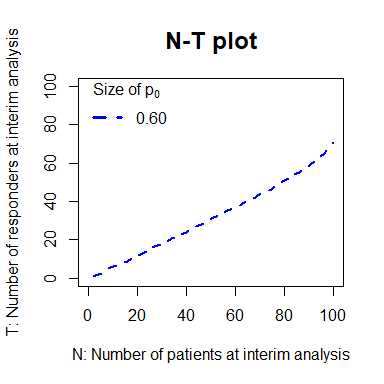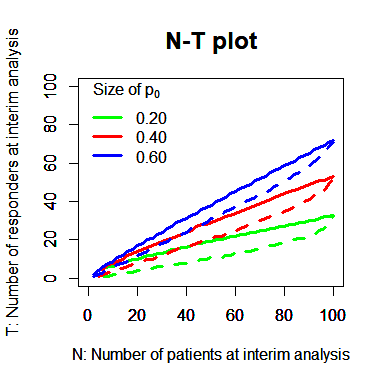# Vignette for IAbin package: Plotting N-T Plane for Decision on Performing an Interim Analysis

## 1. Introduction

In randomized-controlled trials, interim analyses are often planned for possible early termination of the trial for claiming superiority or futility of a new therapy. Such formal interim analyses are performed, closely following the specifications in the study protocol to maintain the overall type I error rate at a nominal level. While unblinding is necessary to conduct the formal interim analysis in blinded studies, data before unblinding also have information, to some extent, about the potential treatment difference between the groups.

We develop a blinded data monitoring tool for the trials measuring binary outcome (especially considering the response rate). We assume that one interim analysis is planned for early termination for superiority or futility of the new treatment, and it can be possibly skipped when our tool suggests that early termination is unlikely based on the blinded data.

The functions in IAbin package give N-T plot, with which the investigators may decide whether or not to skip some of the planned interim analyses when the interim result at that time point unlikely supports early termination of the trial for superiority or futility of the new treatment. This package contains two functions plotNT.sup and plotNT.fut to plot the N-T plane, which is used for expecting the early termination for superiority and futility, respectively.

## 2. N-T plot

### 2.1) Settings

The arguments in the functions are determined in the design stage of a clinical trial, in which the endpoint is a binary, say, response or non-response. Especially, it is assumed that the response rate of the control therapy (p0) is chosen from the sufficient knowledge by the clinical experts. And the function is used for a trial expecting an interim analysis. Here is an example for the settings of the arguments.

p0 = 0.6
M = 100
q = 0.5
alpha1 = 0.01
cp1 = 0.2

p0 is a value of the expected response rate in the control therapy. If the several possible values are considered for the control efficacy, p0 can be a vector, i.e., p0 = c(0.2, 0.3, 0.4). M is an expected total sample size in both new therapy and control arms. q is an allocation ratio of the new therapy arm $$(0 < q < 1)$$. alpha1 is a critical alpha at an interim analysis. cp1 is a critical conditional power at an interim analysis.

Also, the other arguments for graphics are also included in the functions. The default setting is

xlab = "N: Number of patients at interim analysis"
ylab = "T: Number of responders at interim analysis"
col = "blue"
main = "N-T plot"
lty = 1

If p0 is set as a vector, col should be the same length of vector, i.e., col = c("blue", "red", "green"). The default value of lty is set 1 and 2 for plotNT.sup and plotNT.fut, respectively.

### 2.2) For early stopping for superiority

The function plotNT.sup automatically draws a N-T plot for early stopping for superiority. If the length of p0 is plural, it draws several lines on a plane. Monitoring the total number of patients and the total number of respondes with blindness maintained, if the observed $$(N, T)$$ plot is over the drawn line, we expect that the response rate of the new therapy would be significantly higher than the control, with the significance level alpha1. Otherwise, the result of the test unlikely supports early termination of the trial for superiority of the new treatment, and then the investigators will skip the interim analysis.

When printing the function plotNT.sup, it gives a matrix names N, T, Z_score and P_val. The first and second values correspond to the drawn N-T plot. The third one is the expected Z scores and the two sided p-values at the corresponding (N, T), calculated via

$$Z = \frac{\hat{p_1} - p_0}{\widehat{Var(\hat{p_1})}}$$        and         p-value $$= 2(1 - \Phi(Z))$$,

where $$\hat{p_1} = (T - N(1 - q) p_0)/Nq$$, $$\widehat{Var(\hat{p_1})} = N \hat{r} (1 - \hat{r})/(Nq)^2$$, which is a variance estimator of $$\hat{p_1}$$, $$\hat{r} = q\hat{p_1} + (1-q)p_0$$, and $$\Phi$$ is a cumulative distribution function of the standard normal distribution. If the lenght of p0 is plural, it gives a list.

NT_s = plotNT.sup(p0, M, q, alpha1)print(head(NT_s))
##       N  T  Z_score        P_val
## [1,]  2  2      Inf 0.0000000000
## [2,]  4  4      Inf 0.0000000000
## [3,]  6  6      Inf 0.0000000000
## [4,]  8  8      Inf 0.0000000000
## [5,] 10  9 3.162278 0.0015654023
## [6,] 12 11 3.968971 0.0000721838

### 2.3) For early stopping for futility

The function plotNT.fut draws a N-T plot for early stopping for futility. If the observed $$(N, T)$$ plot is under the drawn line, we expect that the conditional power at that time would be less than cp1, and then unblinded analysis will be recommended for early stopping for futility of the new therapy.

When printing the function plotNT.fut, it gives a matrix names N, T, Z_score and CP. CP is the expected conditional power at the corresponding (N, T).

NT_f = plotNT.fut(p0, M, q, alpha1, cp1)### 2.4) For early stopping for superiority and futility with several candidate p0

If the study investigators want to know possibilitiy of early stopping for superiority and futility, overlap two graphs via par(new = T).

NT_s3 = plotNT.sup(p0 = c(0.2, 0.4, 0.6), M, q, alpha1, col = c("green", "red", "blue"))
par(new = T)
NT_f3 = plotNT.fut(p0 = c(0.2, 0.4, 0.6), M, q, alpha1, cp1, col = c("green", "red", "blue"))## 3. Conclusion

The tool serves a useful reference when interpreting the summary of the blinded data during the course of the trial. This can be used to determine whether or not the formal unblinded interim analysis should be conducted, without losing integrity of the study or spending any alpha. This tool will potentially save the study resource/budget by avoiding unnecessary interim analysis.